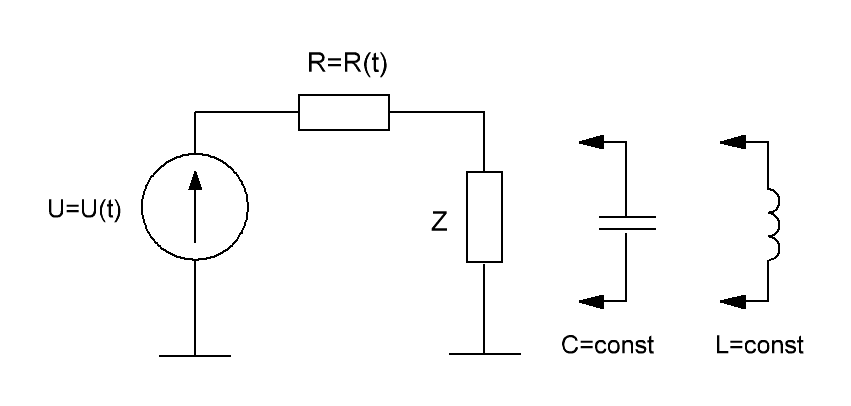Research website of Vyacheslav Gorchilin
2016-07-29
Parametric variation of the resistance, capacitance and inductance in RLC-circuits
It is known that mathematical modeling and analysis of RLC-circuits give a very accurate coincidence with practice. In this work we use this achievement of civilization, and on the example of two different chains will try to prove or disprove some hypotheses more energy by means of parametric changes in resistance, capacitance or inductance.
1. Parametric variation of the resistance in the RC and RL-circuit
Here we consider one approach to solving the search problem of an increase of energy in the RC or RL-circuits, where the resistance $$R$$ can depend on voltage or current in the circuit, or from time to time. This approach is more General than the one here considered previously. To begin with, we generalize the parametric dependence, and present it as: $$R=R(t)$$. As such, it includes all other possible dependencies, because ultimately the whole process runs in time $$t$$.Our electrical circuit consists of a voltage source $$U$$ (with a current source, all can be proved similarly), parametric resistance $$R$$ and the characteristic — $$Z$$, which in reality may be a constant capacitance or inductance. We believe that for the first time in the condenser (in the inductor) the energy is missing.
Capacitive circuit
Write the differential equation for this circuit : $Z\,R(t)\,\dot Y_t + Y = U(t), \quad Y=Y(t) \qquad (1.1)$ where: $$Y$$ — voltage, and $$Z$$ — capacity. We are also aware of a law that changes the current in the tank. Recall it: $\Phi(t) = Z\,\dot Y_t \qquad (1.2)$ where: $$\Phi$$ — current. Substitute the formula (1.2) in (1.1) we get the characteristic equation: $R(t)\,\Phi(t) + Y = U(t) \qquad (1.3)$ Now domnain right and left parts of $$\Phi$$ and printeriem them: $\int_{0}^{T} R(t)\,\Phi(t)^2\, dt + \int_{0}^{T} Y\,\Phi(t)\, dt = \int_{0}^{T} U(t)\,\Phi(t)\, dt \qquad (1.4)$ where: $$T$$ — time of the studied process. In the formula (1.4) the rst term is nothing but the energy dissipated in the resistance $$R$$, the second — potential energy in the capacitor, and to the right of the equal sign is the energy that takes the power source for the whole process.
If the first and the third term is clear, then we need to clarify. Substituting back to the formula (1.2), we obtain this integral: $\int_{0}^{T} Y\,Z\,\dot Y_t\, dt = Z \int_{0}^{T} Y\, dY = \frac{Z}{2} Y^2\, \bigg |_{0}^{T} \qquad (1.5)$ But $$Y(0)$$ is the voltage on the tank for the first time which can be only zero, and hence the desired second term is: $\int_{0}^{T} Y\,\Phi(t)\, dt = \frac{Z}{2} Y(T)^2 \qquad (1.6)$ note that this term can only be positive. Move him to the right side of (1.4) and get our proof: $\int_{0}^{T} R(t)\,\Phi(t)^2\, dt = \int_{0}^{T} U(t)\,\Phi(t)\, dt - \frac{Z}{2} Y(T)^2 \qquad (1.7)$ Now the left — the energy that we receive on resistance, and the right — expended by the power source minus the residual on the tank. It is obvious that the resulting energy can be only less or equal to the energy of the power source.
Inductive circuit
The proof for the inductive circuit is carried out in a similar way. The differential equation is: $R(t)\,Y + Z\,\dot Y_t = U(t), \quad Y=Y(t) \qquad (1.8)$ where: $$Y$$ — circuit current, and $$Z$$ — inductance. The voltage dependence of the current on the record form the same: $\Phi(t) = Z\,\dot Y_t \qquad (1.9)$ only here $$\Phi$$ — voltage across the inductor. The characteristic equation in this case looks like this: $R(t)\,Y + \Phi(t) = U(t) \qquad (1.10)$ Damnosa all its members on $$Y$$ and integrating we get: $\int_{0}^{T} R(t)\,Y^2\, dt + \int_{0}^{T} Y\,\Phi(t)\, dt = \int_{0}^{T} U(t)\,Y\, dt \qquad (1.11)$ the second member of the equation do the same as in a capacitive circuit, which leads us to the final equation: $\int_{0}^{T} R(t)\,Y^2\, dt = \int_{0}^{T} U(t)\,Y\, dt - \frac{Z}{2} Y(T)^2 \qquad (1.12)$ Output from it is similar to the previous case: we get the resistance of the energy can only be less than or equal to the energy of the power source.
Insights
The proof shows that in an RC or RL circuit, where capacitance and inductance are constant, and the resistance is parametric, the gain of energy can not be. Moreover, it does not depend on the nature of parametric dependencies, nor from the selected time range.
Next we will describe a much more complex process with parametric capacity and we find a condition for obtaining a gain of energy in this circuit.